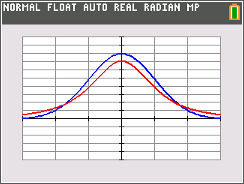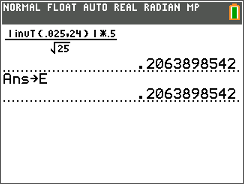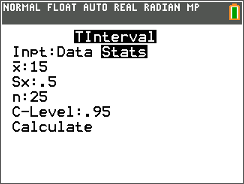••• ##### Device
• TI-84 Plus
• TI-84 Plus Silver Edition
•TI-84 Plus C Silver Edition
•TI-84 Plus CE
• ##### Software

TI Connect™ CE

• ##### Report an Issue

Statistics: Population Mean: σ unknown
by Texas Instruments#### Overview

Students calculate confidence intervals to estimate the true population mean when the standard deviation of the population is not known.

#### Key Steps

•Students will be introduced to the assumptions needed to use the t distribution to estimate the true mean of a population when only the sample mean and standard deviation are known. They will investigate, by graphing, the relationship between a normal distribution and the t distribution.

•Students are presented with a problem to calculate a confidence interval. They will compute the interval, finding the t-value and the error. Then the teacher will lead students in learning how to use the TInterval command for a second method of finding the interval.

•As an extension, students are given a data set and asked to construct three confidence intervals. They will be able to use either method from the activity.